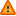You should trust both wikis because the password could be read by the particular administrators.

Clear message

# Sums of Squares used by R in lm, aov and lmer

R calculates sequential or Type I SS by default when using lm, lmer and aov as opposed to the type III SS (default in SPSS) which partials out effects of other terms in the model. A hierarchical approach is suggested which gives the same results for both sums of squares and only looks at the highest order terms in a model.

Type III SS may be obtained using terms of form (for between subjects anova)

```library(car)
fit <- Anova(lm(y~x+z),type=c("III"))```

and for more complex designs with random effects, anova() can be used

```library(nlme)
model.2 <- lme(y~a + b, random=~1|group)
anova(model.2,type="marginal")```

The Anova() procedure can also be used to obtain type 3 sums of squares for lmer in R. The Anova () procedure is available by downloading the car extension package from here and can be inserted into the R library folder located in C:\Program Files\R\R3.x. You can also use the summary() procedure, for obtaining type III SS for lmer from version 2.7.2 of R.

In addition to lm and lme (which requires the nlme extension package), aov may also be used to fit anovas in R.